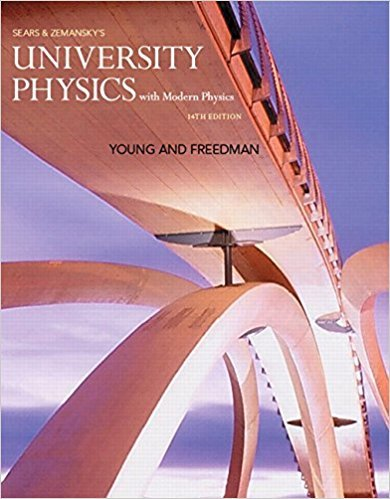×
×

# Answer: Two pieces of clay collide and stick together.ISBN: 9780321973610 228

## Solution for problem Q8.24 Chapter 8

University Physics with Modern Physics (1) | 14th Edition

• Textbook Solutions
• 2901 Step-by-step solutions solved by professors and subject experts
• Get 24/7 help from StudySoup virtual teaching assistantsUniversity Physics with Modern Physics (1) | 14th Edition

4 5 0 309 Reviews
18
2
Problem Q8.24

Two pieces of clay collide and stick together. During the collision, which of these statements are true? (a) Only the momentum of the clay is conserved; (b) only the mechanical energy of the clay is conserved; (c) both the momentum and the mechanical energy of the clay are conserved; (d) the kineticenergy of the clay is conserved

Step-by-Step Solution:
Step 1 of 3

Goals • To learn why a light wave contains both electric and magnetic fields • To relate the speed of light to the fundamental constants of electromagnetism • To describe electromagnetic waves • To determine the power carried by electromagnetic waves • To describe standing electromagnetic waves Introduction • Why do metals reflect light • We will see that light is...

Step 2 of 3

Step 3 of 3

##### ISBN: 9780321973610

The answer to “Two pieces of clay collide and stick together. During the collision, which of these statements are true? (a) Only the momentum of the clay is conserved; (b) only the mechanical energy of the clay is conserved; (c) both the momentum and the mechanical energy of the clay are conserved; (d) the kineticenergy of the clay is conserved” is broken down into a number of easy to follow steps, and 57 words. Since the solution to Q8.24 from 8 chapter was answered, more than 228 students have viewed the full step-by-step answer. This textbook survival guide was created for the textbook: University Physics with Modern Physics (1), edition: 14. The full step-by-step solution to problem: Q8.24 from chapter: 8 was answered by , our top Physics solution expert on 01/09/18, 07:46PM. University Physics with Modern Physics (1) was written by and is associated to the ISBN: 9780321973610. This full solution covers the following key subjects: . This expansive textbook survival guide covers 44 chapters, and 4574 solutions.

Unlock Textbook Solution